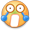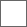### 【17-05-27】闯关得分计算公式S(r, t, m, n) = S1(r, m) + S2(r, t, m) + S3(r, m, n),

S1(r, m) = [ (1+r) [a3 (m-1)^3 + a2 (m-1)^2 + a1 (m-1) + a0] ],
S2(r, t, m) = [ (1+r) [φ(t) S1(r, m)] ],
S3(r, m, n) = [ (1+r) [k S1(r, m)·(n-1)] ].

a3 = 0.07, a2 = 1.2, a1 = 28.85, a0 = 300, k = 0.03;
r 按账号等级 Lv 计算, 早期无等级视为 0 级, 0—59 级的加成率满足
r = [Lv/2 + 1]/100, 60 级的加成率为 0.30;
Lv, m 与 n 均为整数, 分别满足 0 ≤ Lv ≤ 60, 1 ≤ m ≤ 10, 1 ≤ n ≤ 20;

0, 0.2, 0.5, 0.8, 1.0, 1.2, 1.5.#### 扫二维码下载贴吧客户端